Courses

# Test: Arrays, Stack, Queues & Linked List- 3

## 20 Questions MCQ Test Question Bank for GATE Computer Science Engineering | Test: Arrays, Stack, Queues & Linked List- 3

Description
This mock test of Test: Arrays, Stack, Queues & Linked List- 3 for Computer Science Engineering (CSE) helps you for every Computer Science Engineering (CSE) entrance exam. This contains 20 Multiple Choice Questions for Computer Science Engineering (CSE) Test: Arrays, Stack, Queues & Linked List- 3 (mcq) to study with solutions a complete question bank. The solved questions answers in this Test: Arrays, Stack, Queues & Linked List- 3 quiz give you a good mix of easy questions and tough questions. Computer Science Engineering (CSE) students definitely take this Test: Arrays, Stack, Queues & Linked List- 3 exercise for a better result in the exam. You can find other Test: Arrays, Stack, Queues & Linked List- 3 extra questions, long questions & short questions for Computer Science Engineering (CSE) on EduRev as well by searching above.
QUESTION: 1

### A program P reads in 500 integers in the range (0,100) representing the scores of 500 students.If prints the frequency of each score above 50, what would be the best way for P to store the frequencies?

Solution:

There are 500 students the score range is 0 to 100. Print the frequency of those student whose score above 50. So frequency range contains score from 50 to 100, so an array of 50 numbers is suitable for representing the frequency.

QUESTION: 2

### Let A be a square matrix of size nxn. Consider the following pseudocode. What is the expected output?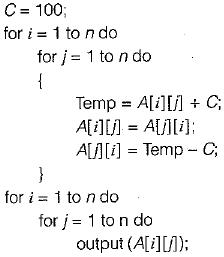Solution: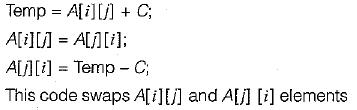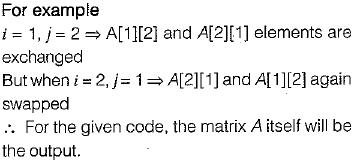QUESTION: 3

### The following sequence of operations is performed on a stack: PUSH (10), PUSH (20), POP, PUSH (10), PUSH (20), POP, POP, POP, PUSH (20), POP  The sequence of values popped out is:

Solution: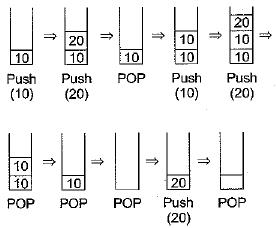∴ The sequence of values popped out is 20, 20, 10, 10, 20.

QUESTION: 4

Which of the following permutations can be obtained in the output (in the same order) using a stack assuming that the input is the sequence 1,2, 3, 4, 5 in that order?

Solution:

PUSH one by one element into Stack and POP accordingly desired output. Only second option can satisfy.

QUESTION: 5

The postfix expression for the infix expression A + B * ( C + D ) / F + D * E is

Solution:

Infix: A + B*(C + D)/F + D*E
Postfix: ABCD + *F/ + DE*++

QUESTION: 6

Consider the following statements:
(i) First-in-first out types of computations are efficiently supported by STACKS.
(ii) Implementing LISTS on linked lists is more efficient than implementing LISTS on an array for almost all the basic LIST operations.
(iii) Implementing QUEUES on a circular array is more efficient than implementing QUEUES, on a linear array with twg indices.
(iv) Last-in-first-out type of computations are efficiency supported by QUEUES.

Solution:

Stack is UFO system so FIFO not supported by stack hence option statement 1 is incorrect and queue is using FIFO so statement 4 is also wrong.

QUESTION: 7

Which of the following is essential for converting an infix expression to the post fix form efficiently?

Solution:

Operands never change its position during expression conversion so only operator stack is needed.

QUESTION: 8

A priority queue Q is used to implement a stack that stores characters. PUSH (C) is implemented INSERT (Q, C, K) where K is an appropriate integer key chosen by the implementation. POP is implemented as DELETEMIN(Q). For a sequence of operations, the keys chosen are in

Solution:

In stack last element will be deleted first but according to question, it should be wprk like queue. So last element deleted at last, for that we give lower priority to last element. So all elements should be in strictly decreasing order.

QUESTION: 9

What value would the following function return for the input x - 95?
Function fun (x: integer): integer;
Begin
If x > 100 then fun = x - 10
Else fun := fun(fun (x + 11))
End;

Solution: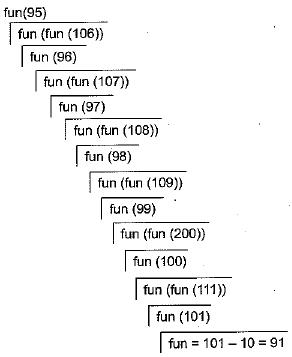So the return value is 91.

QUESTION: 10

The best data structure to check whether an arithmetic expression has balanced parentheses is a

Solution:

The stack is a data structure in which each push (insert) or pop (delete) operation takes constant 0(1) time. The best data structure to check whether an arithmetic expression has a balanced parentheses.
Step 2: Process each char of an expression string.
Step 3: If it is open, push it on the stack.
Step 4: If it is closed, check it against the top of stack element.
Step4.1: If stack empty or not a matching parentheses then not balanced and return false.
Step 4.2: Otherwise it matches, pop the open parentheses then goto step 2.
Step 5: If stack is empty at the end, return true.
Step 6: If not empty then some parentheses is unmatched, return false.

QUESTION: 11

A circularly linked list is used to represent a Queue. A single variable p is used to access the Queue. To which node should p point such that both the operations enQueue and deQueue can be performed in constant time?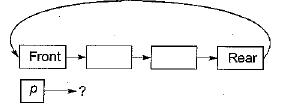Solution: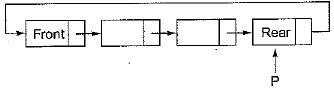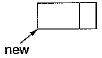new → next = Rear → next;
Rear → next = new;
Rear = new;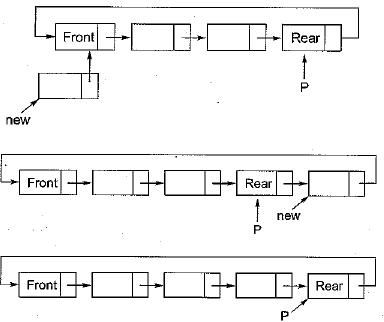It will take constant time.
Similarly delete node from Front code will be:
Front = Rear → next;
Rear → next = Front → next;
Free (Front);
It will also take constant time.

QUESTION: 12

Assume that the operators + , -, x are left associative and ^ is right associative. The order of precedence (from highest to lowest) is ^, x, +, The postfix expression corresponding to the infix expression a + b x c - d ^ e ^ f is

Solution:

According to conditions given:
⇒ [(a + (b x c)) - (d ^ (e ^ f))]
⇒ [(a + ( b c x)) - (d ^ (e f ^))]
⇒ [(abc x +) - (def^^)]
⇒ abc x + def^^ -

QUESTION: 13

A program attempts to generate as many permutations as possible of the string, ‘abcd' by pushing the characters a, b, c, d in the same order onto a stack, but it may pop off the top character at any time. Which one of the following strings CANNOT be generated using this program?

Solution:

• For every element push an element and pop immediately i.e. push (a) then pop will give permutation abed.
• For all elements push (a), push (b), push (c) and push (d) then pop, pop, pop, pop will give us deba.
• Push (a), push (b), push (c) then pop, pop, pop will give is eba, then push (d) and pop will gives us deba.
• Option (d) is not possible because a cannot pop after c and before ‘b’.

QUESTION: 14

The following postfix expression with single digit operands in evaluated using a stack
8 2 3 ^ / 2 3* + 5 1 * -
Note that A is the exponentiation operator. The top two elements of the stack after the first* is evaluated are

Solution:

Given postfix expression is
8 2 3 ^ / 23 * + 5 1 * -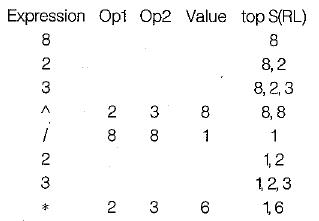So the top two elements of the stack are 6, 1 after the first * is evaluated.

QUESTION: 15

Suppose a stack implementation supports an instruction REVERSE, which reverses the order of elements on the stack, in addition to the PUSH and POP instructions. Which one of the following statements is TRUE with respect to this modified stack? .

Solution:

Consider an queue with elements inserted in same order as element present in queue.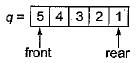Now to implement stack same as queue: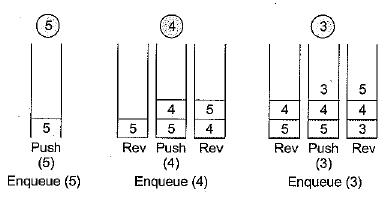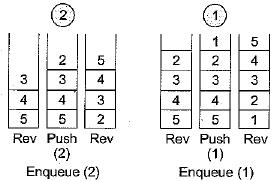A queue can be implemented where ENQUEUE takes a sequence of three instructions and DEQUEUE takes a single instruction.

QUESTION: 16

The result evaluating the postfix expression 10 5 + 60 6/* 8 - is

Solution:

10 5 + 60 6 / * 8 -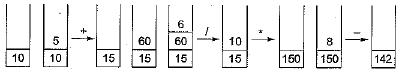Result is 142.

QUESTION: 17

In a circular linked list organization, insertion of a record involves modification of

Solution: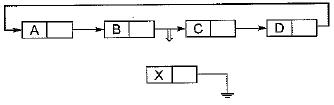In above linked list a new record X wili be inserted in between B and C.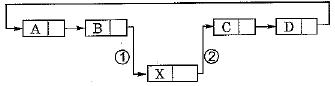Two pointers are modified to insert X record.

QUESTION: 18

Linked lists are not suitable data structures of which one of the following problems?

Solution:

Using linked list binary search wili take O(n) time. So binary search is inefficient with linked list.

QUESTION: 19

In the worst case, the number of comparisons needed to search singly linked list of length n for a given element is

Solution:

Linear search number of comparisons: O(n).

QUESTION: 20

Consider the function f defined below: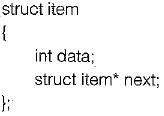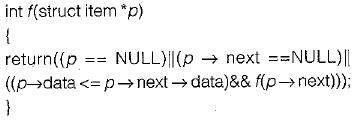For a given linked list p, the function f returns 1 if and only if

Solution:

For a given linked list p, the function f returns 1 if and only if the eiements in the list are stored in non-decreasing (increasing) order of the data value.
f returns 1 if p == NULL or p Next == NULL or p → data < = p → next data, satisfies in linked list for every node p.